# 手把手实现一个HTML模板引擎

1,097

``````<span>Message: {{ msg }}</span>
``````

1. `<% ... %>` 可以包裹一个 JavaScript 语句：
``````<%for ( let i = 0; i < 10; i++ ) { %>
<% console.log(i) %>
<% } %>
``````
1. `<%= ... %>` 可以获取当前执行环境下的变量：

``````const render = template('<div><%= data.name %></div>');
console.log(render({name: 'hi'})) // <div>hi</div>
``````

``````// @filename: webpack.config.js
plugins: [
new HtmlWebpackPlugin({
title: 'Custom template',
// Load a custom template (lodash by default)
template: 'index.html'
})
]

// @filename: index.html
<!DOCTYPE html>
<html>
<meta charset="utf-8"/>
<title><%= htmlWebpackPlugin.options.title %></title>
<body>
</body>
</html>
``````

ps: 下面讲解的代码其实就是 underscorejs 的 `_.template` 的思路，只不过略过了对一些边界情况的兼容。

``````<!DOCTYPE html>
<html lang="en">

<meta charset="UTF-8">
<title></title>

<body>
<script id="templ" type="text/html">
<%for ( var i = 0; i < data.list.length; i++ ) { %>
<li>
<a data-id="<%= data.list[i].id %>">
<%=data.list[i].name %>
</a>
</li>
<% } %>
</script>
<script src="./template.js"></script>
</body>

</html>
``````

``````let content = document.querySelector('#templ').innerHTML
``````

``````const print = new Function('str', 'console.log(str)')
print('hello world') // hello world
``````

``````const print = function (str) {console.log(str)}
``````

``````new Funtion('data', x);
``````

``````<%for ( var i = 0; i < data.list.length; i++ ) { %>
<li>
<a data-id="<%= data.list[i].id %>">
<%=data.list[i].name %>
</a>
</li>
<% } %>
``````

1. 使用正则 `/<%=([\s\S]+?)%>/g` 匹配到 `<%= ... %>` 格式的字符串
2. 使用正则 `/<%([\s\S]+?)%>/g` 匹配到 `<% ... %>` 格式的字符串

``````content = content.replace(/<%=([\s\S]+?)%>/g, function(_, evaluate) {
return "'+\n" + evaluate + "+\n'"
})
``````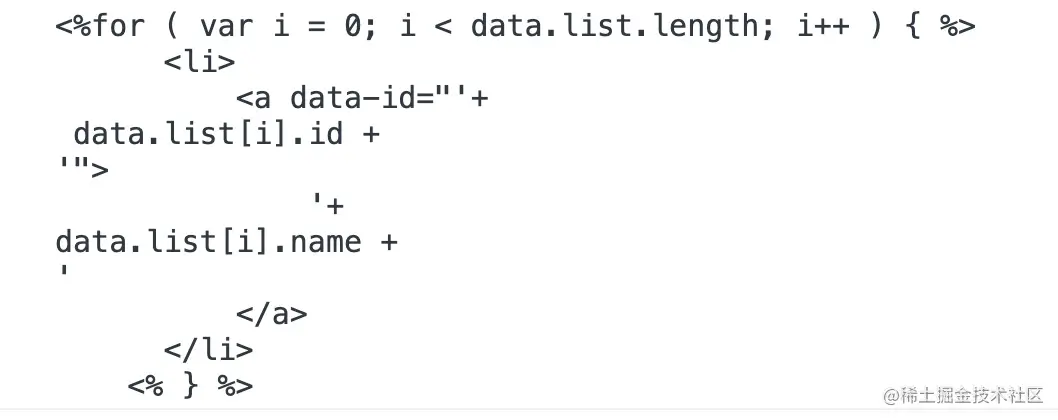``````content = content.replace(/<%([\s\S]+?)%>/g, function(match,interpolate) {
return "';\n" + interpolate + "\n_p +='";
})
``````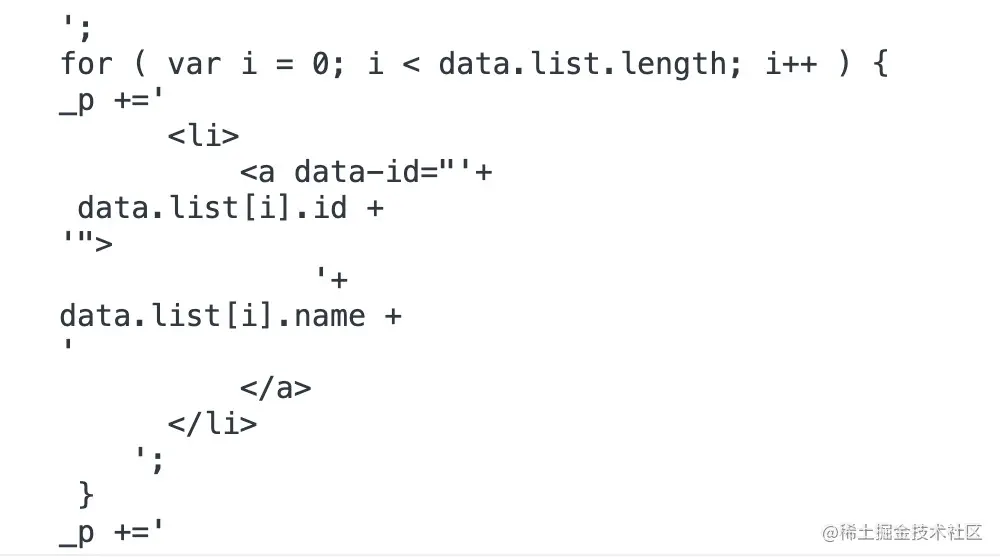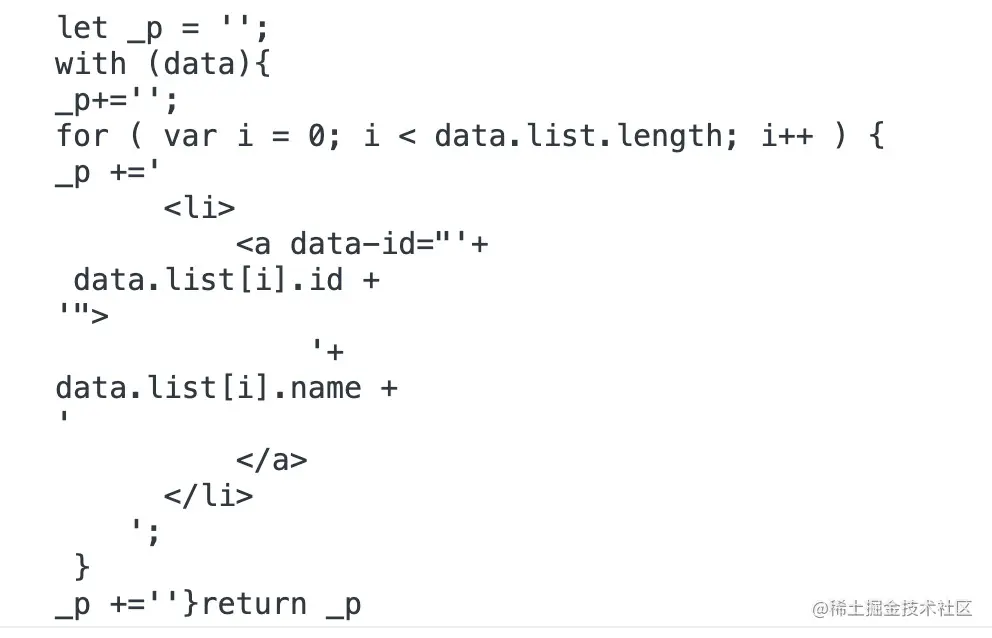``````const a = 1;
const b = 2;
``````

`\n` 一样的还有下面几个，列一个统一的表：

'\`
\\\
\r\\r
\n\\n
\u2028\\u2028
\u2029\\u2029

``````var escapes = {
"'": "'",
'\\': '\\',
'\r': 'r',
'\n': 'n',
'\u2028': 'u2028',
'\u2029': 'u2029'
};

var escapeRegExp = /\\|'|\r|\n|\u2028|\u2029/g;

function escapeChar(match) {
if (match === "'") {
return "\'"
}
return '\\' + escapes[match];
}
``````

``````function escapeChar(match) {
return '\\' + escapes[match];
}
``````

``````byte[] byteArray1 = "\\".getBytes();
byte[] byteArray2 = "\\\\".getBytes();
System.out.println(byteArray1) // 
System.out.println(byteArray2) // [92, 92]
``````

``````content = content.replace(escapeRegExp, function(match) {
return escapeChar(match);
}
``````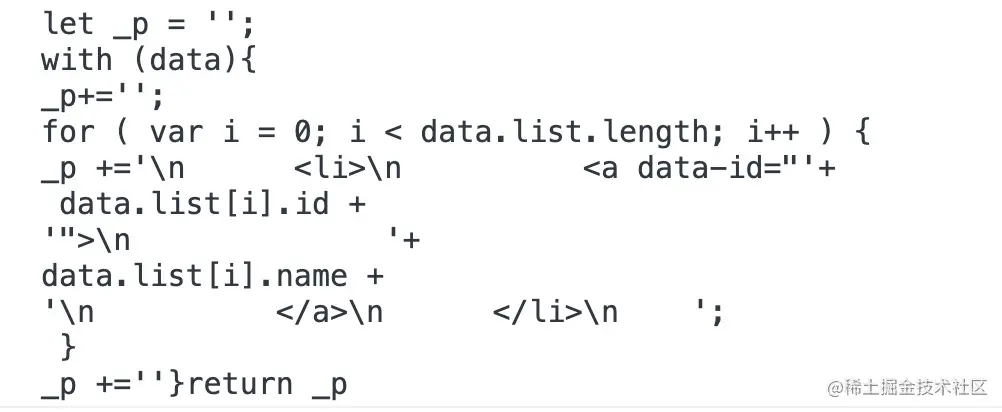``````const render = new Function('data', content);
``````

``````render({
list: {name: 'Bob', id: 1}
})
``````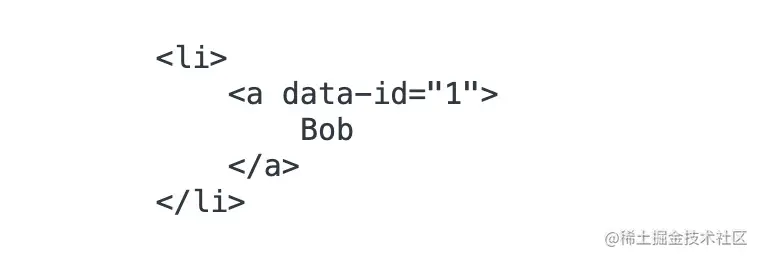underscore 的思路也是这样子，只不过，它做的更简洁。

underscore 只需要遍历一遍，碰到 `<%= ... %>`或者 `<% ... %>` 后，先把上一次匹配结果的结束到这次匹配结果之前的特殊字符处理掉，然后再判断当前匹配到的模板语法怎么处理，依次迭代，直到匹配到字符串结尾。

``````var interpolate = '123';
var __t;
(__t= (interpolate)) == null ? '' : __t
``````

``````interpolate == null ? interpolate : ''
``````

## 完整代码

``````<!-- @filename: index.html -->
<!DOCTYPE html>
<html lang="en">

<meta charset="UTF-8">
<title></title>

<body>
<script id="templ" type="text/html">
<%for ( var i = 0; i < data.list.length; i++ ) { %>
<li>
<a data-id="<%= data.list[i].id %>">
<%=data.list[i].name %>
</a>
</li>
<% } %>
</script>
<script src="./index.js"></script>
</body>

</html>
``````
``````// @filename: main.js
let content = document.querySelector('#templ').innerHTML

var settings = {
evaluate: /<%([\s\S]+?)%>/g,
interpolate: /<%=([\s\S]+?)%>/g,
};

var escapes = {
"'": "'",
'\\': '\\',
'\r': 'r',
'\n': 'n',
'\u2028': 'u2028',
'\u2029': 'u2029'
};

var escapeRegExp = /\\|'|\r|\n|\u2028|\u2029/g;

function escapeChar(match) {
if (match === "'") {
return '\\`'
}
return '\\' + escapes[match];
}

function template(text) {
var matcher = RegExp([
(settings.interpolate || noMatch).source,
(settings.evaluate || noMatch).source
].join('|') + '|\$', 'g');

var index = 0;
var source = "__p+='";
text.replace(matcher, function (match, interpolate, evaluate, offset) {
source += text.slice(index, offset).replace(escapeRegExp, escapeChar);
index = offset + match.length;

if (interpolate) {
source += "'+\n((__t=(" + interpolate + "))==null?'':__t)+\n'";
} else if (evaluate) {
source += "';\n" + evaluate + "\n__p+='";
}

return match;
});
source += "';\n";

var argument = 'data';
source = 'with('+ argument + '||{}){\n' + source + '}\n';

source = "var __t,__p='';" +
source + 'return __p;\n';

var render;
try {
render = new Function(argument, source);
} catch (e) {
e.source = source;
throw e;
}

var template = function (data) {
return render.call(this, data);
};

return template;
}

const render = template(content);

var list = [
{name: 'Bob', id: 1},
{name: 'Jack', id: 2},
]

console.log(render({
list
}))
``````